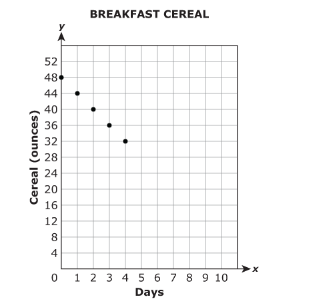Damien W.

# Interpreting Key Features

Ciara has a bowl of cereal for breakfast. The amount of cereal in the box (y) is determined by the number of days (x) that have passed since she purchased the cereal.Note: The graph below shows only a portion of this linear relationship

What is the domain of the function? What does it represent in the context of the problem?

What is the rate of change? What does it represent in the context of the problem?

What is the x-intercept? What does it represent in the context of the problem?

What is the y-intercept? What does it represent in the context of the problem?

Over which interval, if any, is the function positive? What does it represent in the context of the problem?

Over which interval, if any, is the function negative? What does it represent in the context of the problem?